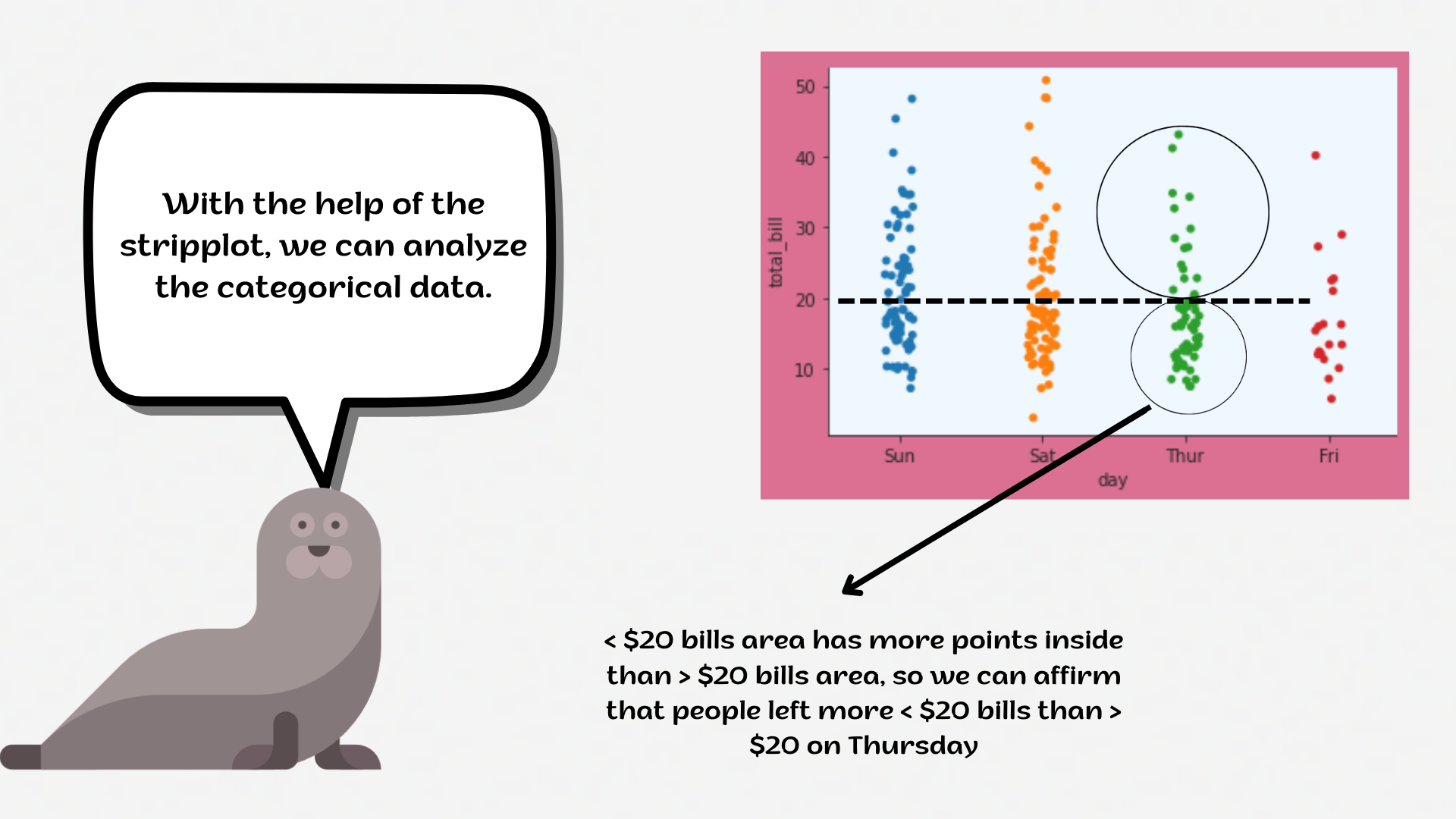Course Content

# Deep Dive into the seaborn Visualization

1. Light Start

2. Distributions of Data

3. Categorical Plot Types

4. Matrix Plots

5. Multi-Plot Grids

6. Regression Models

Deep Dive into the seaborn Visualization

##StripplotIn statistics, a categorical variable is a variable that can take on one of a limited and usually fixed number of possible values, assigning each individual or other unit of observation to a particular group or nominal category on the basis of some qualitative property.

A `stripplot` is a single-axis scatter plot that is used to visualize the distribution of many individual one-dimensional values. The values are plotted as dots along one unique axis, and the dots with the same value can overlap.1. Set the `'whitegrid'` style with the `'aliceblue'` `axes.facecolor`.
2. Create the `stripplot` using the `seaborn` library:
• Set the `x` parameter equals the `'day'`;
• Set the `y` parameter equals the `'total_bill'`;
• Set the `hue` parameter equals the `'smoker'`;
• Set the `size` parameter equals `20`;
• Set the `'crest'` palette;
• Set the `'D'` marker;
• Set the `alpha` parameter equals `0.25`.

Everything was clear?

Section 3. Chapter 1